# Visualisation

Below are examples of plots produced using functions within the visualisation module: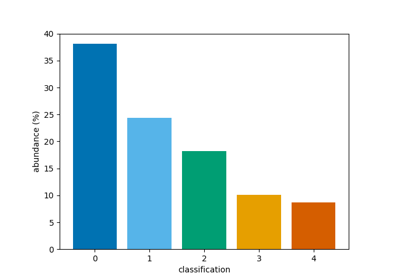Plot a bar chart of classifications

Plot a bar chart of classifications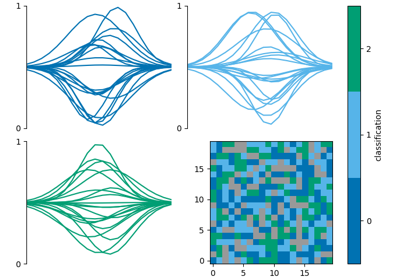Combine multiple classification plots

Combine multiple classification plots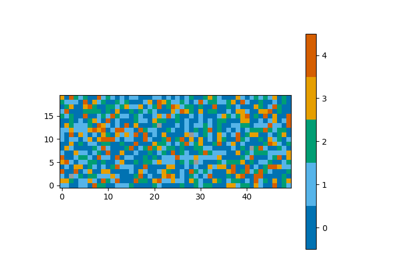Plot a map of classifications

Plot a map of classifications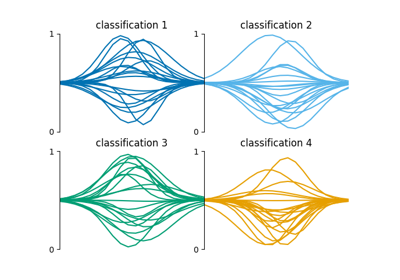Plot a grid of spectra grouped by classification

Plot a grid of spectra grouped by classification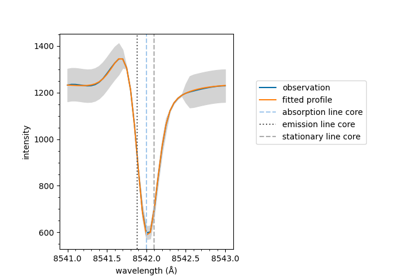Plot a fitted spectrum

Plot a fitted spectrum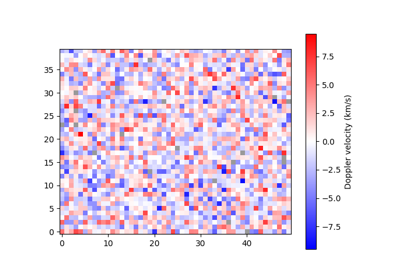Plot a map of velocities

Plot a map of velocities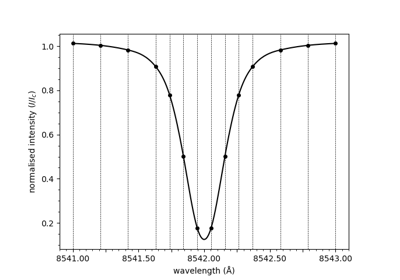Plot a spectrum

Plot a spectrum